Question

Assume you wish to evaluate the risk and return behaviors associated with various combinations of assets V and W under three assumed degrees of​ correlation: perfect​ positive, uncorrelated, and perfect negative. The following average return and risk values were calculated for these​ assets:

 Asset Average​ Return, r Risk​ (Standard Deviation), s V 7.9​% 4.6​% W 12.7​% 9.7​%

a. If the returns of assets V and W are perfectly positively correlated​ (correlation coefficient = + 1​), describe the range of​ (1) return and​ (2) risk associated with all possible portfolio combinations.

b. If the returns of assets V and W are uncorrelated​ (correlation coefficient = 0​), describe the approximate range of ​(1​) return and​ (2) risk associated with all possible portfolio combinations.

c. If the returns of assets V and W are perfectly negatively correlated​ (correlation coefficient = - 1​), describe the range of​ (1) return and​ (2) risk associated with all possible portfolio combinations.

• Return of Portfolio (Rp)

It is weighted average of individual stock return in a portfolio. Two-Assets Portfolio Return formula provided below -

Where,

W = Weight of Stock

R = Return of Stock

• Standard Deviation(risk) of Portfolio ()

It measures the risk of Portfolio. Risk of two assets Portfolio formula provided below-

• Possible Portfolio Combination

It refers to portfolio on optimal portfolio frontier which means each portfolio provide higher return for higher level of risk. portfolio having low return for high risk should be rejected.

1.

Please refer to following spreadsheet and graph for Return and risk of Possible Portfolio combination having stock V and W perfectly positive correlated.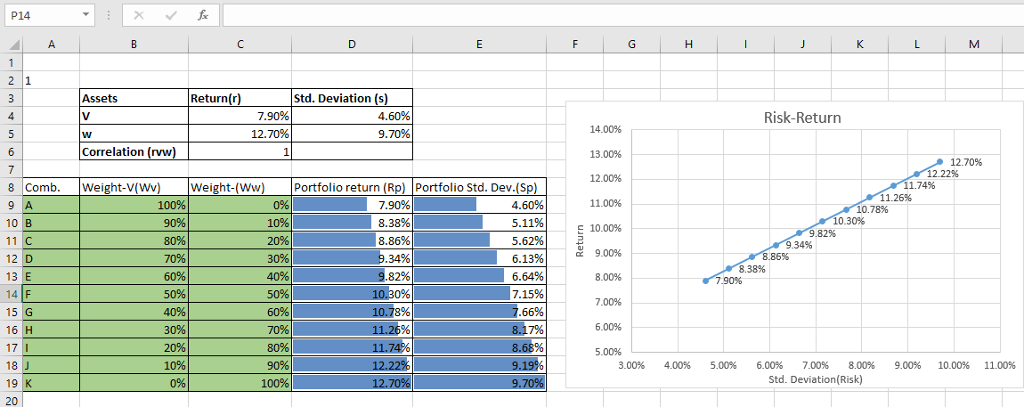Formula Reference -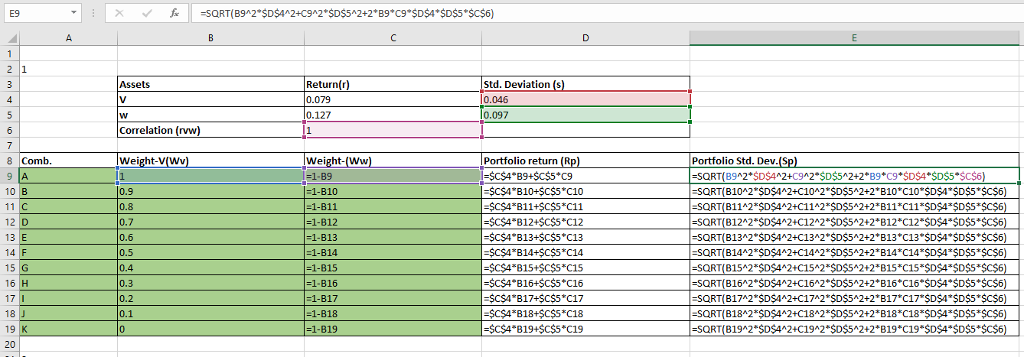We can see in above calculation and graph, when V and W are perfectly positive correlated then all the combination A to Z are optimal which means there is always higher return for higher risk. combination highlighted in green are optimal.

2.

Please refer to following spreadsheet and graph for Return and risk of Possible Portfolio combination having stock V and W uncorrelated.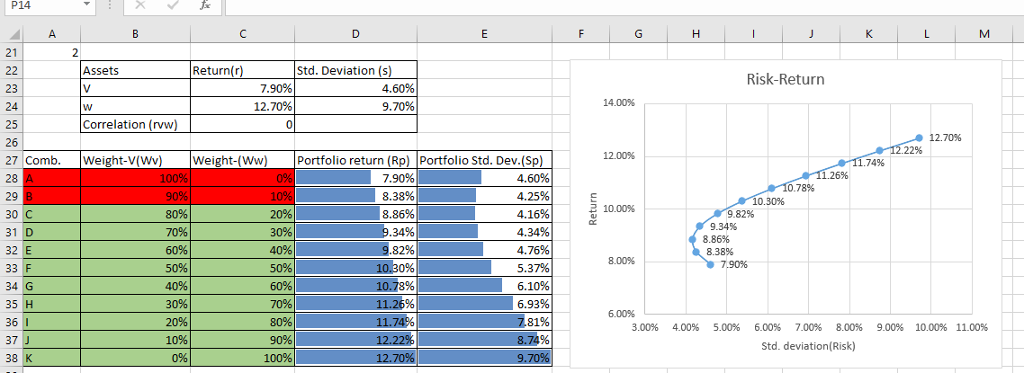Formula Reference -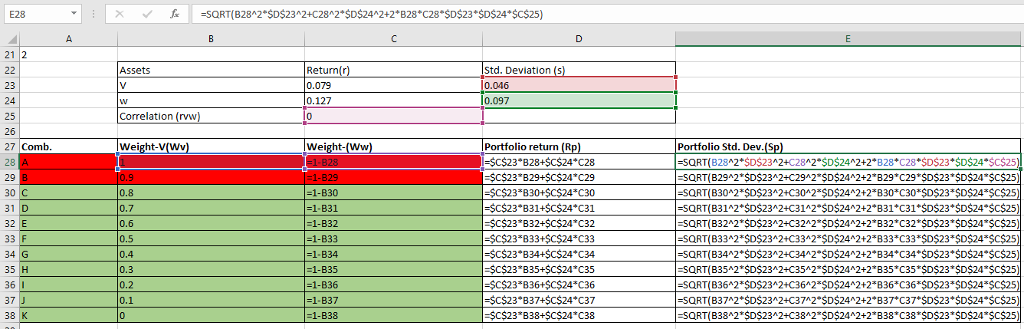We can see in above calculation and graph, when V and W are uncorrelated then only combination C to Z are optimal .and combination A & B which are highlighted in red is not optimal which it has lower return for higher risk.

3.

Please refer to following spreadsheet and graph for Return and risk of Possible Portfolio combination having stock V and W perfectly negative correlated.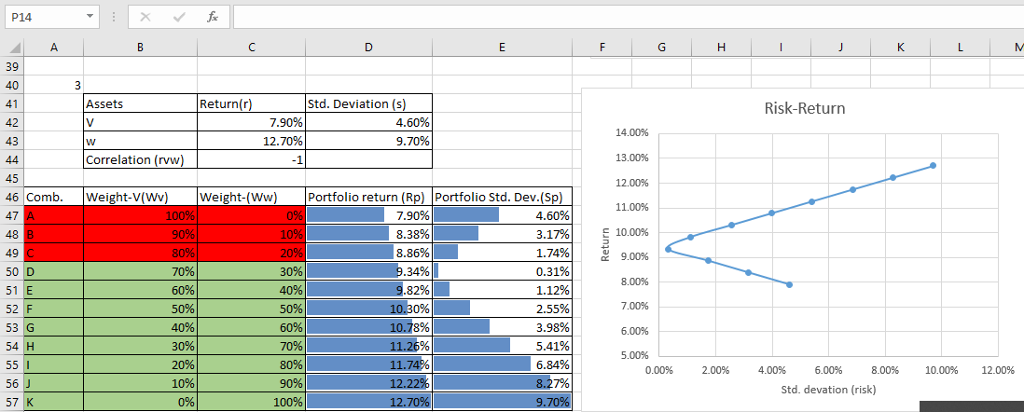Formula Reference -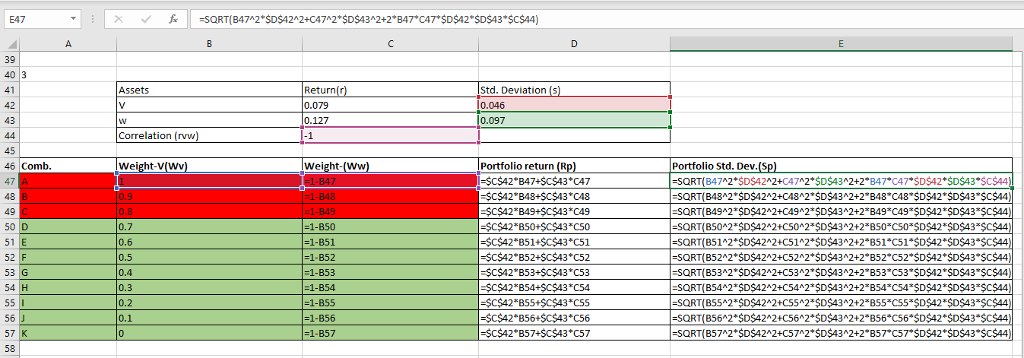Combination highlighted in green D to Z are optimal and combination A,B&C highlighted in red are not optimal.

#### Earn Coins

Coins can be redeemed for fabulous gifts.

Similar Homework Help Questions
• ### LG4 5-14 Correlation, risk, and return Matt Peters wishes to evaluate the risk and return behaviors...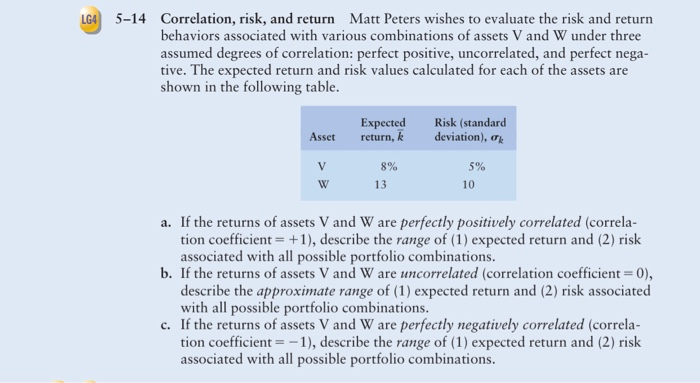LG4 5-14 Correlation, risk, and return Matt Peters wishes to evaluate the risk and return behaviors associated with various combinations of assets V and W under three assumed degrees of correlation: perfect positive, uncorrelated, and perfect nega- tive. The expected return and risk values calculated for each of the assets are shown in the following table. Asset Expected return, k Risk (standard deviation), V 5% 8% 13 a. If the returns of assets V and W are perfectly positively correlated...

• ### Assume you wish to evaluate the risk and return behaviors associated with various combinations of two​ stocks, Alpha Sof...

Assume you wish to evaluate the risk and return behaviors associated with various combinations of two​ stocks, Alpha Software and Beta​ Electronics, under three possible degrees of​ correlation: perfect​ positive, uncorrelated, and perfect negative. The average return and standard deviation for each stock appears​ here: Asset   Average Return,overbar r                   Risk (Standard Deviation), s Alpha   5.1%   30.3% Beta                    11.2%      50.5% a. If the returns of assets Alpha and Beta are perfectly positively correlated​ (correlation coefficient equals plus 1​),...

• ### Correlation, risk, and return Matt Peters wishes to evaluate the risk and return behaviors associated with...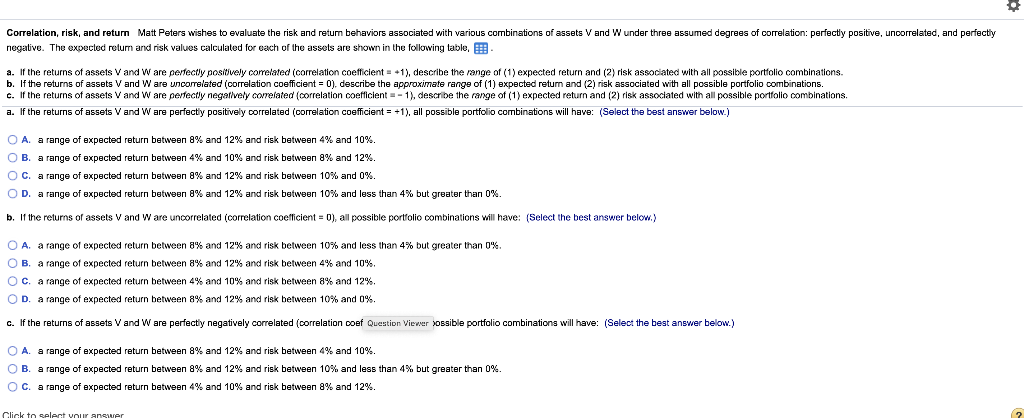Correlation, risk, and return Matt Peters wishes to evaluate the risk and return behaviors associated with various combinations of assets V and W under three assumed degrees of correlation: perfectly positive, uncorrelated, and perfectly negative. The expected return and risk values calculated for each of the assets are shown in the following table, B a. If the returns of assets V and W are perfectly positively correlated correlation coefficient = +1), describe the range of (1) expected return and (2)...

• ### le three alternatives. c. Use your findings in parts a and b to calculate the coefficient of variatio each of the three...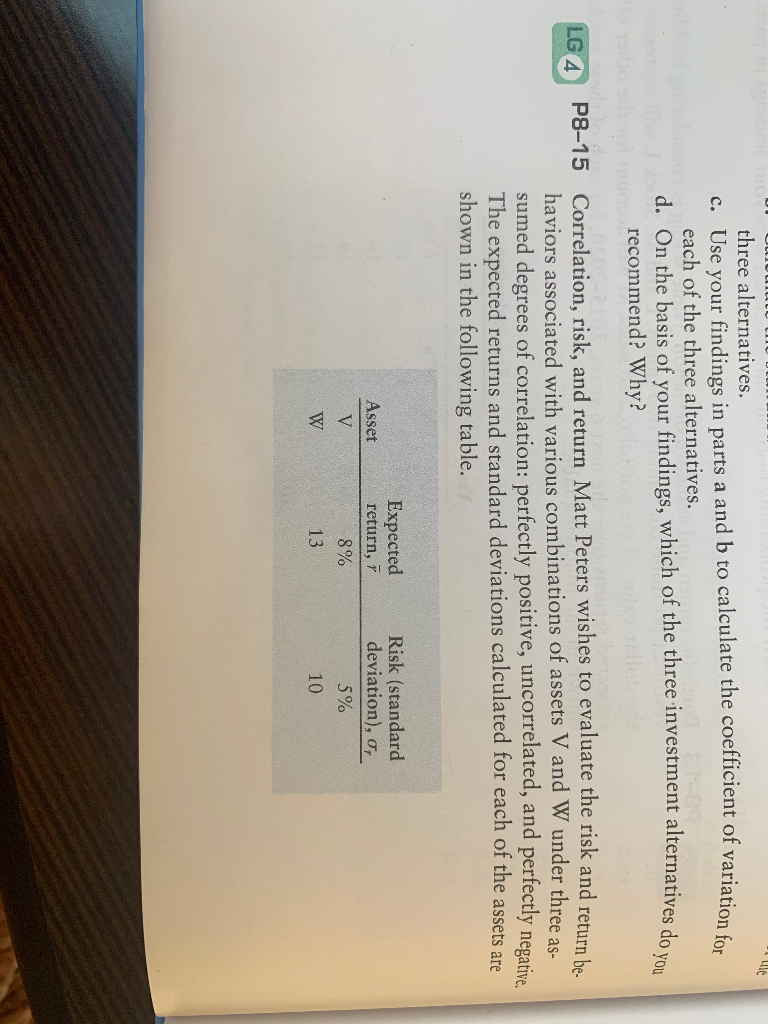le three alternatives. c. Use your findings in parts a and b to calculate the coefficient of variatio each of the three alternatives. d. On the basis of your findings, which of the three investment alternatives do recommend? Why? LG 4 P8-15 Correlation, risk, and return Matt Peters wishes to evaluate the risk and return be haviors associated with various combinations of assets V and W under three as- sumed degrees of correlation: perfectly positive, uncorrelated, and perfectly negative The...

• ### p8-15 A-C 333 CHAPTER 8 Risk and Return a. If the returns of assets V and...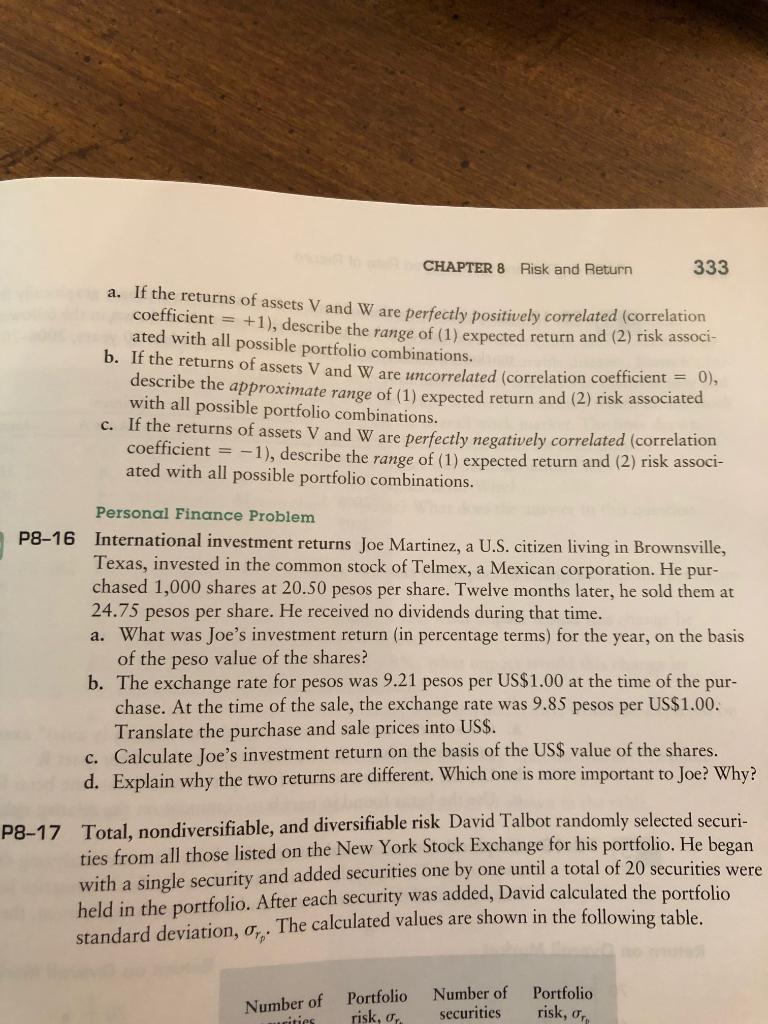p8-15 A-C 333 CHAPTER 8 Risk and Return a. If the returns of assets V and W are perfectly positively correlated (correlation coefficient = +1), describe the range of (1) expected return and (2) risk associ- ated with all possible portfolio combinations. b. If the returns of assets V and W are uncorrelated (correlation coefficient = 0), describe the approximate range of (1) expected return and (2) risk associated with all possible portfolio combinations c. If the returns of assets...

• ### 2. 3: Risk and Rates of Return: Risk in Portfolio Context Risk and Rates of Return:...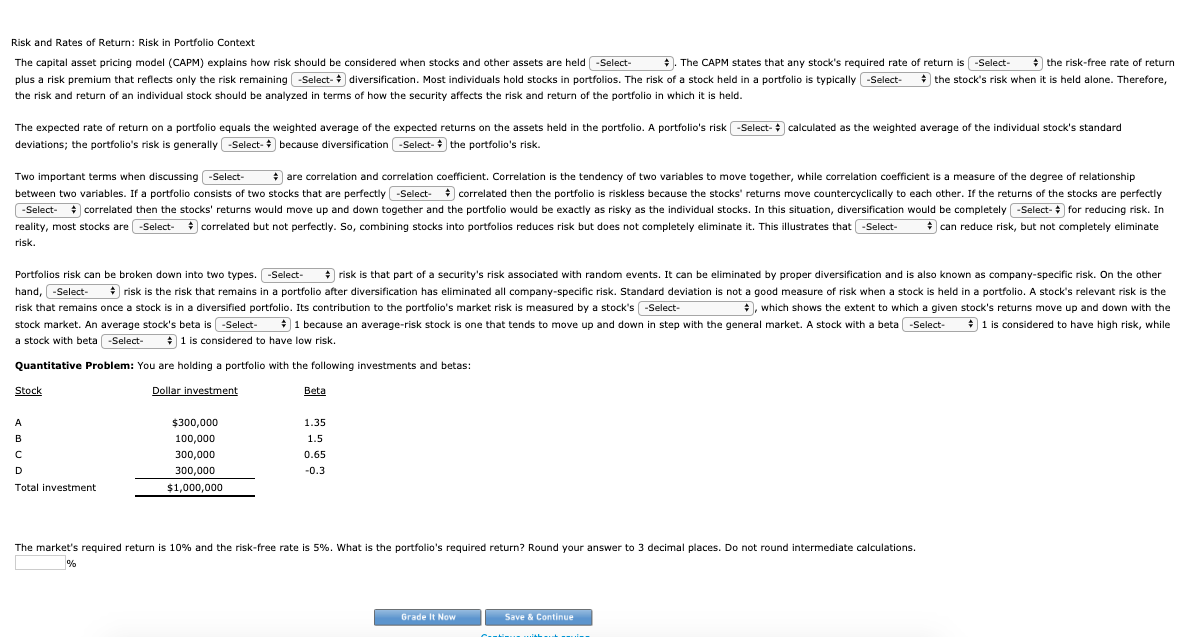2. 3: Risk and Rates of Return: Risk in Portfolio Context Risk and Rates of Return: Risk in Portfolio Context The capital asset pricing model (CAPM) explains how risk should be considered when stocks and other assets are held . The CAPM states that any stock's required rate of return is the risk-free rate of return plus a risk premium that reflects only the risk remaining diversification. Most individuals hold stocks in portfolios. The risk of a stock held in...

• ### Term Answer Description Risk A. The potential for variability in the possible outcomes associated with an...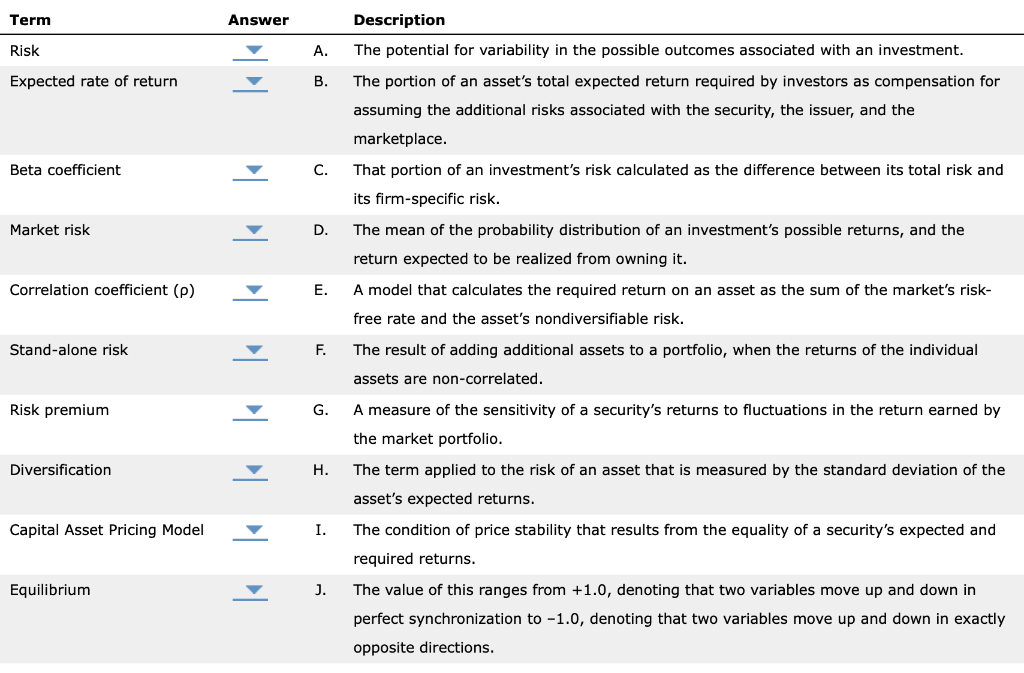Term Answer Description Risk A. The potential for variability in the possible outcomes associated with an investment. Expected rate of return The portion of an asset's total expected return required by investors as compensation for assuming the additional risks associated with the security, the issuer, and the marketplace. Beta coefficient That portion of an investment's risk calculated as the difference between its total risk and its firm-specific risk. Market risk The mean of the probability distribution of an investment's possible...

• ### od The capital asset pricing model (CAPM) explains how risk should be considered when stocks and...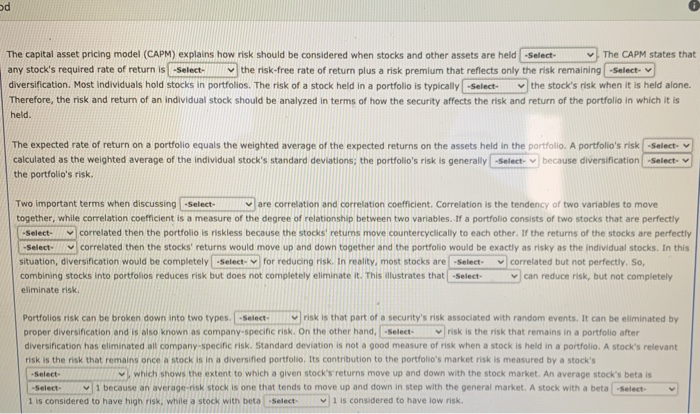od The capital asset pricing model (CAPM) explains how risk should be considered when stocks and other assets are held -Select- The CAPM states that any stock's required rate of return is -Select the risk-free rate of return plus a risk premium that reflects only the risk remaining -Select- diversification. Most individuals hold stocks in portfolios. The risk of a stock held in a portfolio is typically -Select the stock's risk when it is held alone. Therefore, the risk and...

• ### Ch 02: Assignment - Risk and Return: Part 1 Term Answer Risk A Expected rate of...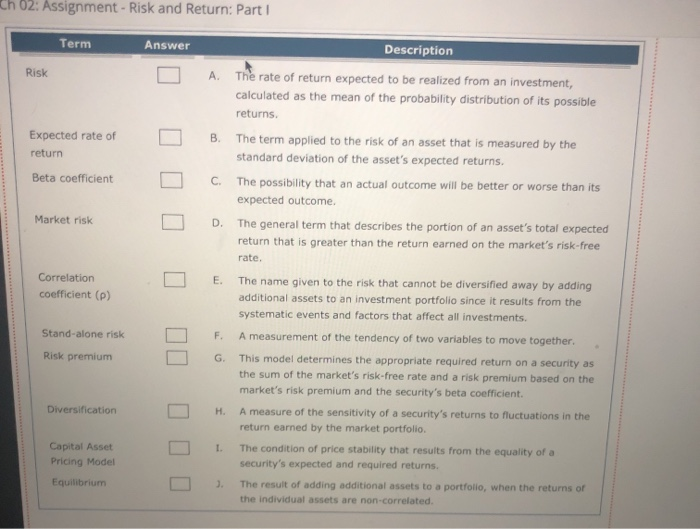Ch 02: Assignment - Risk and Return: Part 1 Term Answer Risk A Expected rate of return B Description The rate of return expected to be realized from an investment, calculated as the mean of the probability distribution of its possible returns. The term applied to the risk of an asset that is measured by the standard deviation of the asset's expected returns. The possibility that an actual outcome will be better or worse than its expected outcome The general...

• ### 1. Perfectly ________ correlated series move exactly together and have a correlation coefficient of ________, while perf...

1. Perfectly ________ correlated series move exactly together and have a correlation coefficient of ________, while perfectly ________ correlated series move exactly in opposite directions and have a correlation coefficient of ________. A. negatively; -1; positively; +1 B. negatively; +1; positively; -1 C. positively; -1; negatively; +1 D. positively; +1; negatively; -1 2. If two assets having perfectly negatively correlated returns are combined in a portfolio, then some combination of those two assets will ________. A. have more risk than...